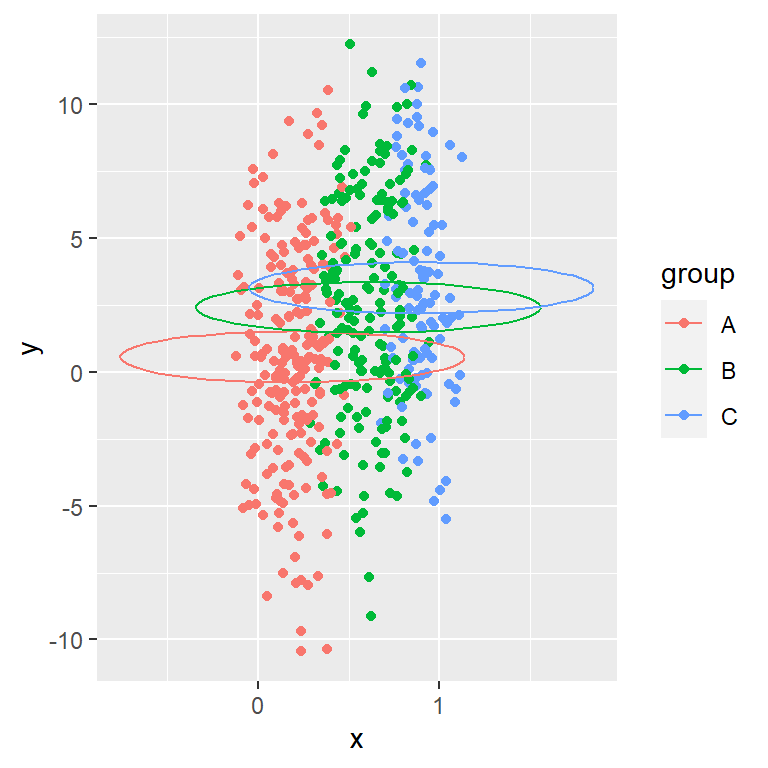# Scatter plot with ellipses in ggplot2

## Sample data

Consider the following data frame which consists on two numerical variables and a grouping variable with three different groups for this tutorial.

``````set.seed(2)

# Data simulation
x <- runif(500)
y <- 4 * x ^ 2 + rnorm(length(x), sd = 4)
group <- ifelse(x < 0.4, "A",
ifelse(x > 0.8, "C", "B"))
x <- x + runif(length(x), -0.15, 0.15)

# Data frame
df <- data.frame(x = x, y = y, group = group)``````

## Adding ellipses with `stat_ellipse`

Default ellipse

You can add an ellipse to your scatter plot adding the `stat_ellipse` layer, as shown in the example below.

``````# install.packages("ggplot2")
library(ggplot2)

ggplot(df, aes(x = x, y = y)) +
geom_point() +
stat_ellipse()``````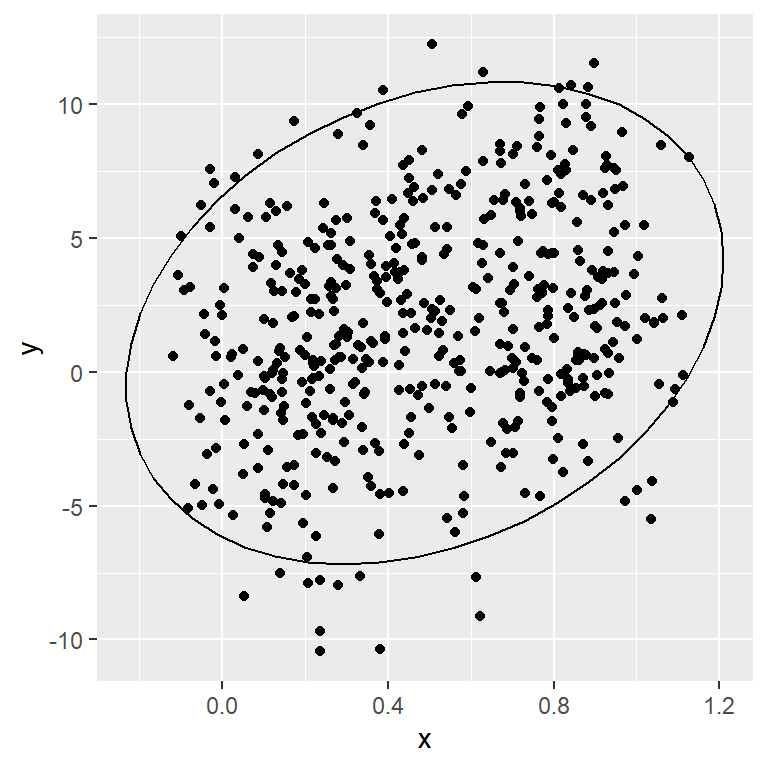Customization

The color, line type and line width of the ellipse can be customized with `color`, `linetype` and `lwd` arguments, respectively.

``````# install.packages("ggplot2")
library(ggplot2)

ggplot(df, aes(x = x, y = y)) +
geom_point() +
stat_ellipse(color = 2,
linetype = 2,
lwd = 1.2)``````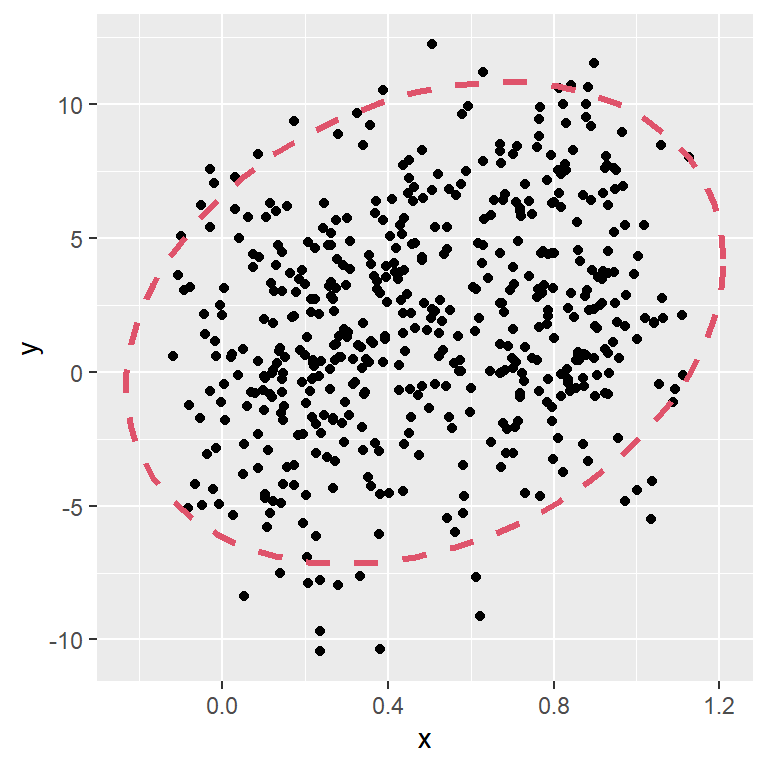Confidence levels

By default, the `stat_ellipse` function draws a 95% confidence level for a multivariate t-distribution. You can modify this level with `level` argument.

``````# install.packages("ggplot2")
library(ggplot2)

ggplot(df, aes(x = x, y = y)) +
geom_point() +
stat_ellipse(level = 0.9) +
stat_ellipse(level = 0.95, color = 2) +
stat_ellipse(level = 0.99, color = 3)``````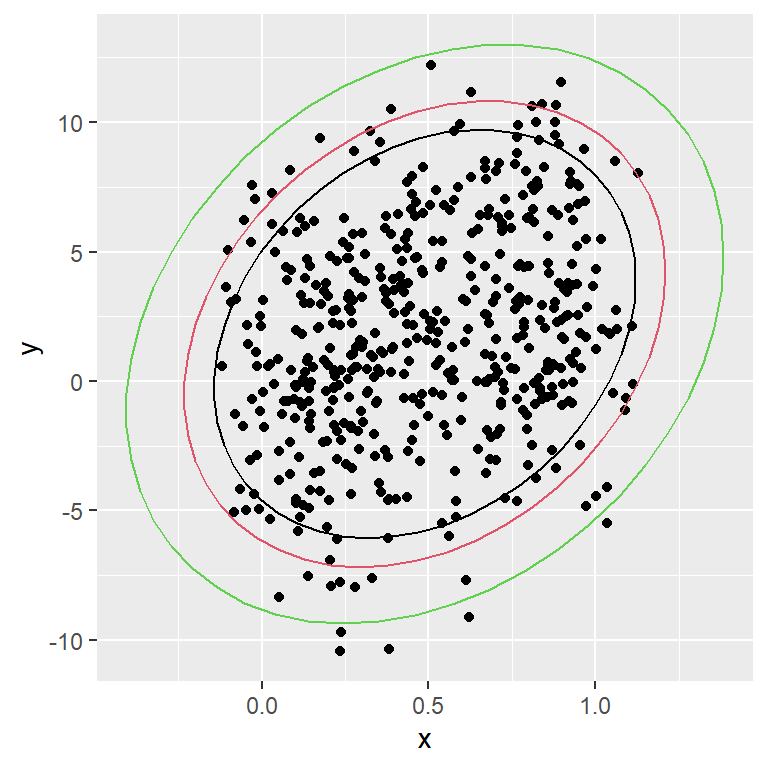Segments

The default number of segments to create the ellipse is 51, but you can modify this number with `segments` argument.

``````# install.packages("ggplot2")
library(ggplot2)

ggplot(df, aes(x = x, y = y)) +
geom_point() +
stat_ellipse(segments = 10)``````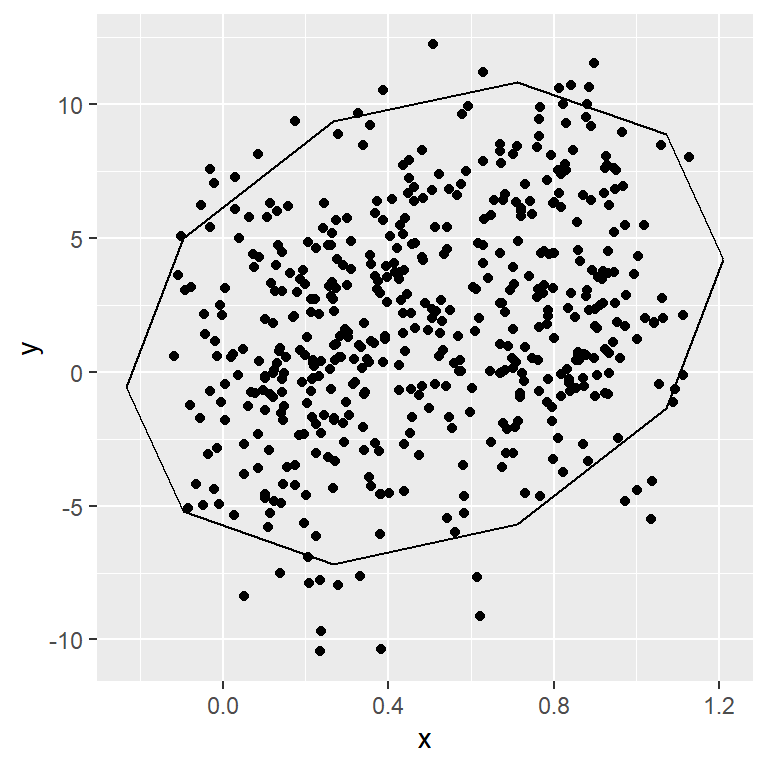Ellipses by group

When you create a scatter plot by group, the ellipses are created for each group.

``````# install.packages("ggplot2")
library(ggplot2)

ggplot(df, aes(x = x, y = y, color = group)) +
geom_point() +
stat_ellipse()``````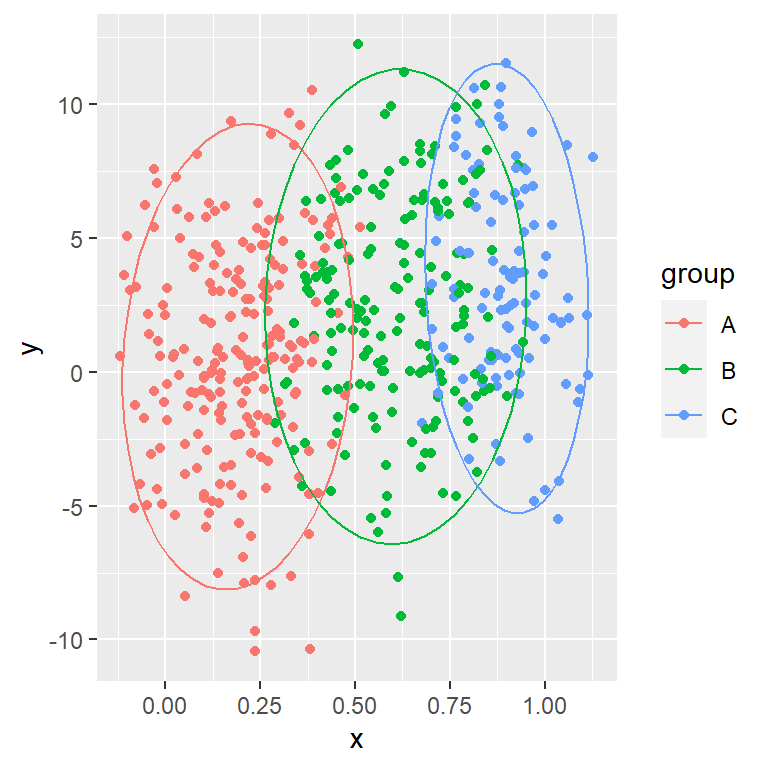Linetype by group

You can also change the line type of the ellipses based on the group, passing the grouping variable to the `linetype` argument of the `aes` function.

``````# install.packages("ggplot2")
library(ggplot2)

ggplot(df, aes(x = x, y = y, color = group,
linetype = group)) +
geom_point() +
stat_ellipse()``````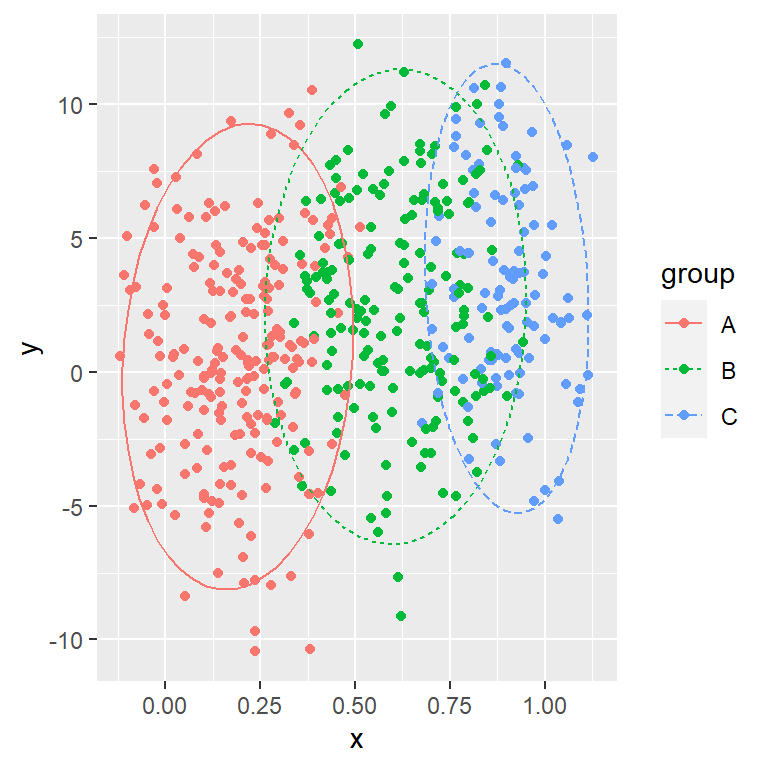## Filling the area of the ellipses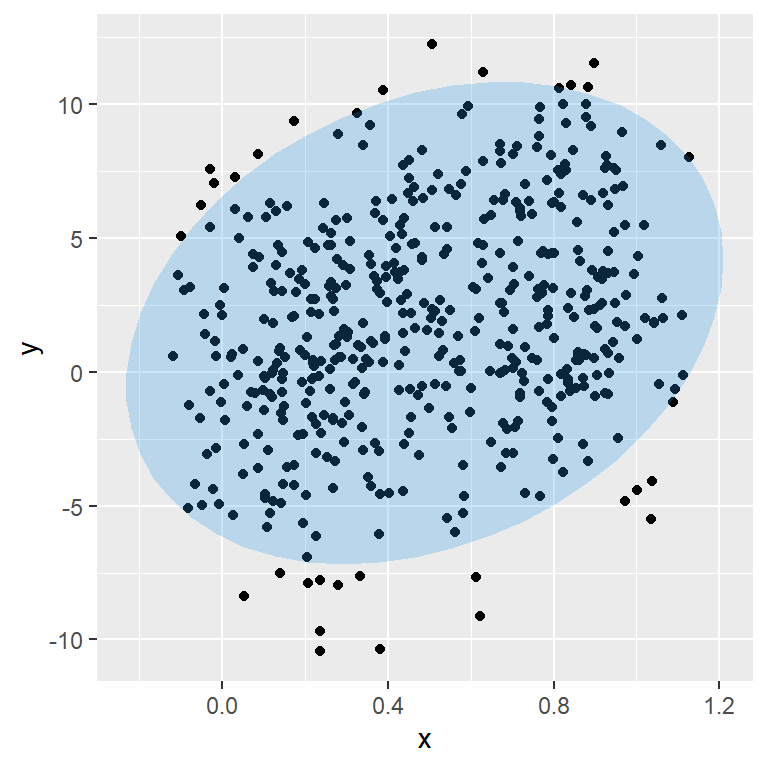Polygon

The `stat_ellipse` uses `geom_path` by default to create the ellipse, but if you set `geom = "polygon"` a polygon will be created. Note that you can change the level of transparency with `alpha`.

``````# install.packages("ggplot2")
library(ggplot2)

ggplot(df, aes(x = x, y = y))+
geom_point() +
stat_ellipse(geom = "polygon",
fill = 4, alpha = 0.25)``````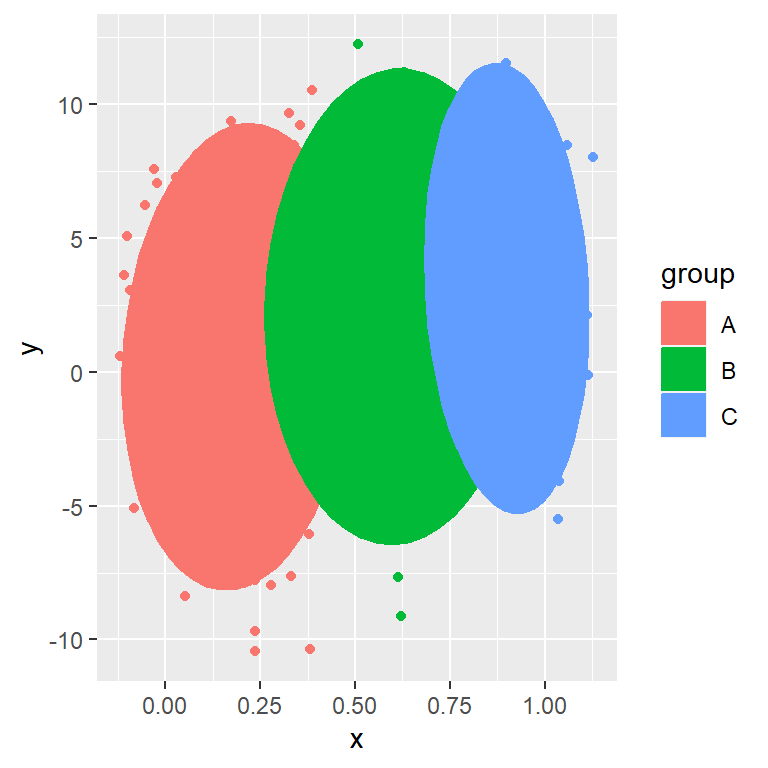Polygon by group

If you create a scatter plot by group and set `geom = "polygon"` inside `stat_ellipse` the polygons areas will be filled by group.

``````# install.packages("ggplot2")
library(ggplot2)

ggplot(df, aes(x = x, y = y, color = group)) +
geom_point() +
stat_ellipse(geom = "polygon",
aes(fill = group))``````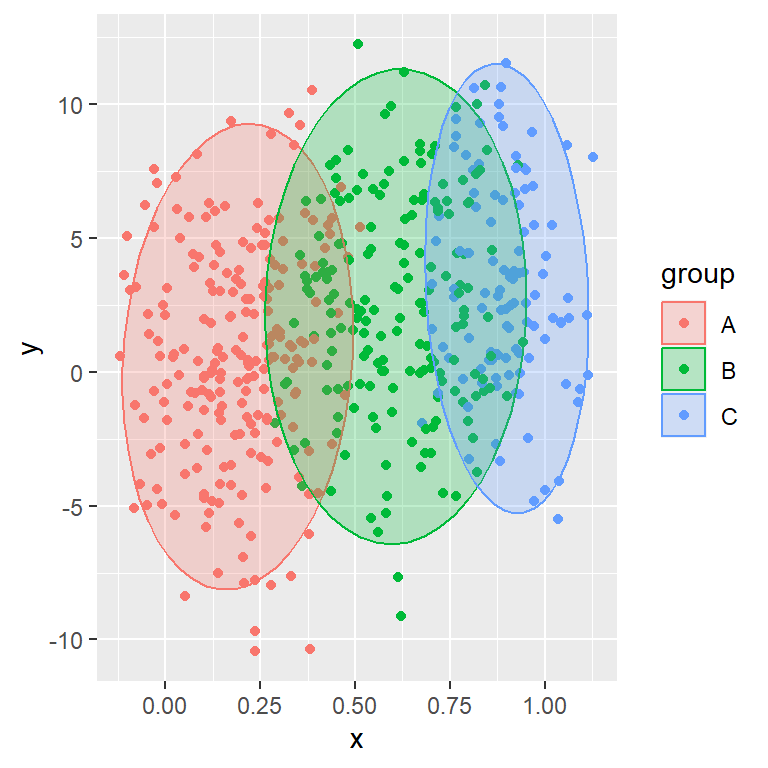Polygon by group with transparency

As in one of the previous examples, the `alpha` argument can be used to set the level of transparency of the polygons.

``````# install.packages("ggplot2")
library(ggplot2)

ggplot(df, aes(x = x, y = y, color = group)) +
geom_point() +
stat_ellipse(geom = "polygon",
aes(fill = group),
alpha = 0.25)``````

## Ellipse types

Normal ellipse

By default, the `stat_ellipse` function assumes a multivariate t-distribution (`type = "t"`). However, you can set `type = "norm"` to assume a multivariate normal distribution.

``````# install.packages("ggplot2")
library(ggplot2)

ggplot(df, aes(x = x, y = y, color = group)) +
geom_point() +
stat_ellipse(type = "t") +
stat_ellipse(type = "norm", linetype = 2)``````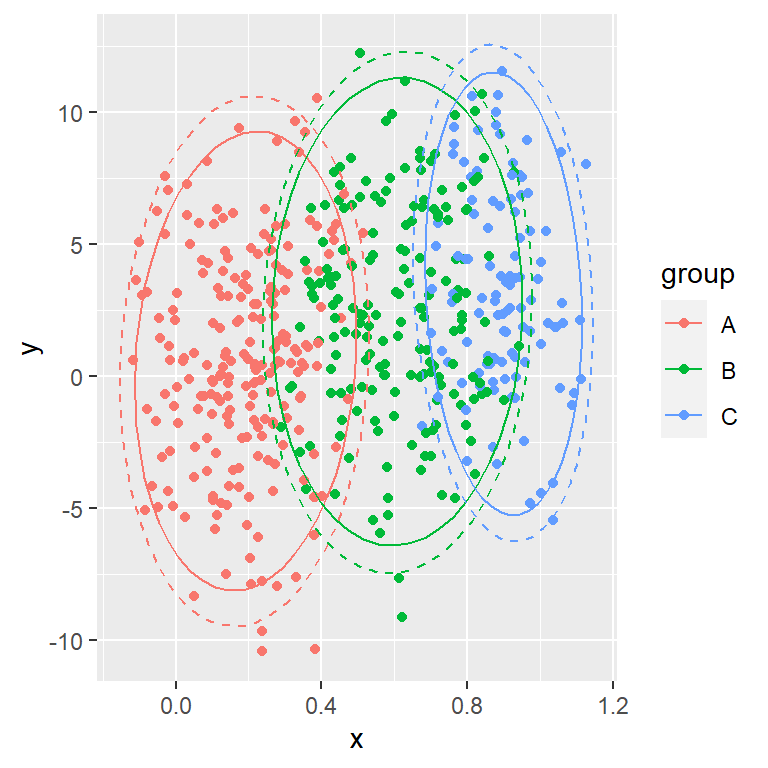Euclidean ellipse

The other option is setting `type = "euclid"` for an euclidean ellipse. Note that the ellipse won’t appear circular unless you set `coord_fixed`. In this scenario, if you set a level, the level will be the radius of the circle to be drawn.

``````# install.packages("ggplot2")
library(ggplot2)

ggplot(df, aes(x = x, y = y, color = group)) +
geom_point() +
stat_ellipse(type = "euclid")``````